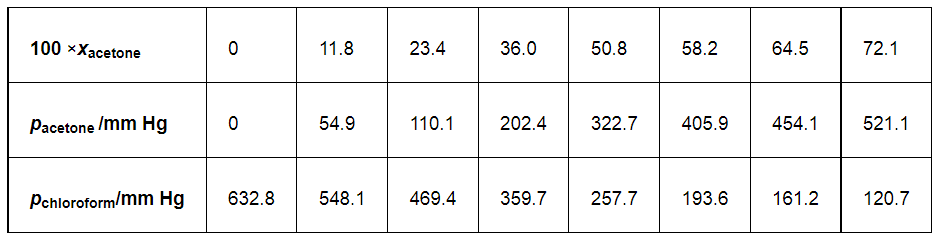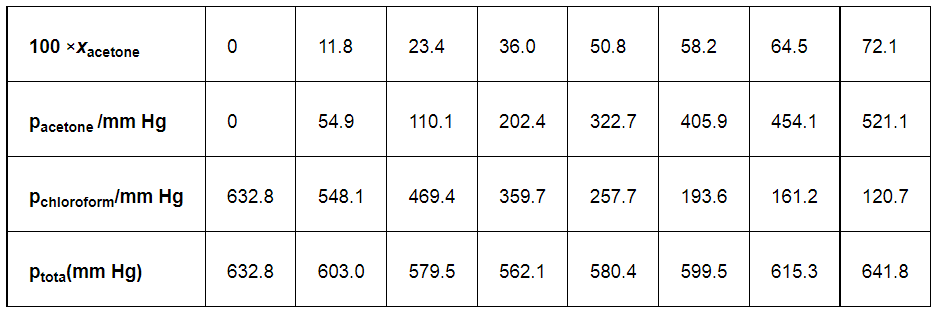# Vapour pressure of pure acetone and chloroform at 328 K are 741.8 mm Hg and 632.8 mm Hg respectively.Question:

Vapour pressure of pure acetone and chloroform at 328 K are 741.8 mm Hg and 632.8 mm Hg respectively. Assuming that they form ideal solution over the entire range of composition, plot ptotal’ pchloroform’ and pacetone as a function of xacetone. The experimental data observed for different compositions of mixture is.Solution:

From the question, we have the following dataIt can be observed from the graph that the plot for the ptotal of the solution curves downwards. Therefore, the solution shows negative deviation from the ideal behaviour.